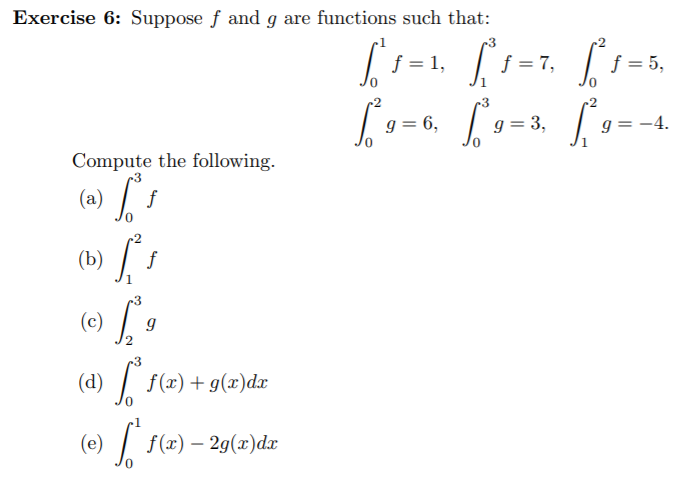# Exercise 6: Suppose f and g are functions such that:-3f = 1, i=7,f = 5,-3Г»g = 6,g = 3,:-4.Compute the following.(a)f(b) / .-3(c)-3(d) / f(x)+g(æ)dx(e)f(«) — 29(х)dx

Question
16 viewshelp_outlineImage TranscriptioncloseExercise 6: Suppose f and g are functions such that: -3 f = 1, i=7, f = 5, -3 Г» g = 6, g = 3, :-4. Compute the following. (a) f (b) / . -3 (c) -3 (d) / f(x)+g(æ)dx (e) f(«) — 29(х)dx fullscreen
check_circle

Step 1

Suppose f and g are functions such that

Step 2
Step 3

### Want to see the full answer?

See Solution

#### Want to see this answer and more?

Solutions are written by subject experts who are available 24/7. Questions are typically answered within 1 hour.*

See Solution
*Response times may vary by subject and question.
Tagged in

### Other